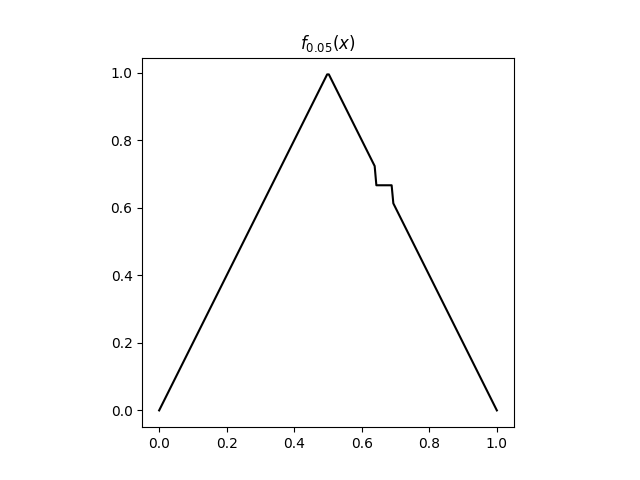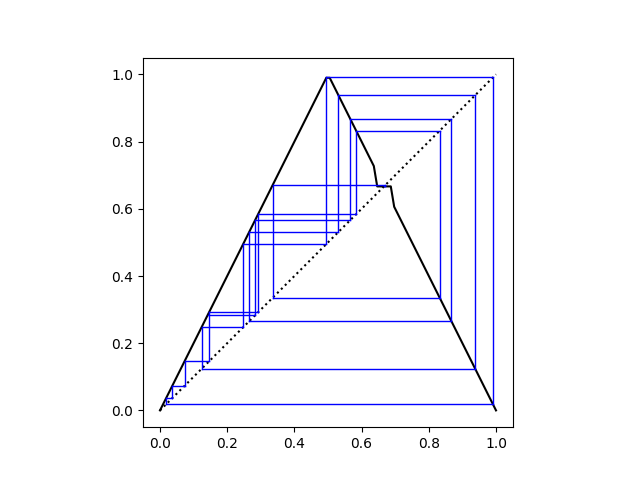# Adding a tiny trap to stop chaos

The tent map is a famous example of a chaotic function. We will show how a tiny modification of the tent maps retains continuity of the function but prevents chaos.

The tent map is the function f: [0, 1] → [0, 1] defined byThis map has an unstable fixed point at x0 = 2/3.  If you start exactly at x0, then you’ll stay there forever as you keep iteratively applying f. But if you start a tiny bit away from x0, you’ll move away from your starting point and bounce around throughout the interval [0, 1].

Now pick some positive ε less than 1/6  and modify the function f on the interval

I =[x0 – ε, x0 + ε]

as described in . To do this, we define fε to be the same as f outside the interval I. We then create a flat spot in the middle of the interval by defining fε to be x0 on

[x0 – ε/2, x0 + ε/2]

and extend fε linearly on the rest of I.

Here’s a plot of fε with ε = 0.05.Now here’s a cobweb plot of the iterates of f0.05 starting at π – 3.The iterates of fε always converge to x0 in finite time. If the iterates ever wander into the interval [x0 – ε/2, x0 + ε/2]  then they get trapped at x0. And because the tent map is ergodic, nearly all sequences will wander into the entrapping interval.

Here’s Python code to make the construction of fε explicit.

    def tent(x):
if x < 0.5:
return 2*x
else:
return 2*(1 - x)

def interpolate(x, x1, y1, x2, y2):
"Linearly interpolate with f(x1) = y1 and f(x2) = y2"
m = (y2 - y1)/(x2 - x1)
return y1 + m*(x - x1)

def tent2(x, epsilon):
x0 = 2/3
if x < x0 - epsilon or x > x0 + epsilon:
return tent(x)

x1 = x0 - epsilon/2
x2 = x0 + epsilon/2

if x > x1 and x < x2:
return x0

return interpolate(x, x1, tent(x1), x2, tent(x2))


## Revenge of floating point

In theory, almost all starting points should lead to sequences that converge to x0 in finite time. But it took a fair amount of trial and error to come up the plot above that illustrates this. For most starting points that I tried, the sequence of iterates converged to 0.

Now 0 is an unstable fixed point. This is easy to see: if x ever gets close to 0, the next iterate is 2x, twice as far from 0. Iterates keep getting doubled until they're larger than 1/2. How can this be?

Computers can only represent fractions with some power of 2 in the denominator. And I believe the tent map (not the modified tent map with a trap) will always converge to 0 when starting with a floating point number.

Here are the iterates of the tent map starting at (the floating point representation of) π - 3:

0 0.28318530717958623
1 0.5663706143591725
2 0.8672587712816551
3 0.26548245743668986
...
29 0.13050460815429688
30 0.26100921630859375
31 0.5220184326171875
32 0.955963134765625
33 0.08807373046875
34 0.1761474609375
35 0.352294921875
36 0.70458984375
37 0.5908203125
38 0.818359375
39 0.36328125
40 0.7265625
41 0.546875
42 0.90625
43 0.1875
44 0.375
45 0.75
46 0.5
47 1.0
48 0.0

Note that the sequence doesn't sneak up on 0: it leaps from 1 to 0. So this does not contract the argument above that points near zero are repelled away.

Related posts: More on dynamical systems

 Why less than 1/6? So we stay in the same branch of the definition of f. The distance from x0 to 1/2 is 1/6.

 Achim Clausing, Ducci Matrices. American Mathematical Monthly, December 2018.

## 4 thoughts on “Adding a tiny trap to stop chaos”

1. The discreteness of floating point representation effectively creates little flat spots everywhere, in particular around zero.

The line x=y passes through the flat spot near zero. It’s also possible but less certain that, at least in some floating point representations, that the x=y line passes though the flat spot at 2/3.

2. Jonathan

> 45 0.75
> 46 0.5
> 47 1.0

> Note that the sequence doesn’t sneak up on 0: it leaps from 1/2 to 0

That looks like a leap to 1.0?

This reminds me of another way to “break” chaos by changing a very small neighborhood, which uses the Rokhlin Lemma. In particular, begin with an invertible, aperiodic measure-preserving dynamical system (mpds); for example, the irrational rotation map defined by T(x) = x+a mod 1. The measure (we can think of it as “length” with our example) should total to 1 across the whole system. We assume it’s aperiodic–that is, the set of all periodic point is measurable negligible–and wish to show that there exists a *totally* periodic system S which exists on the same set and agrees with T on a set of measure 1-epsilon.

The Rokhlin Lemma allows us to claim that we can effectively view such a MPDS as a sort of long, thin “laminar flow” with a tiny chaotic region. (At least, I think of it as a laminar flow ) In particular, for any n and epsilon we can find some measurable set E such that the first n image sets E, TE, T^2E, … T^(n-1)E are all pairwise disjoint sets and they cover all of the system except for a measure epsilon subset; you can think of these sets as cross-sections of a garden hose, with the faucet at E and the spout at T^(n-1)E, where most of the system’s chaos (from our view) is concentrated. Well, how do you make such a system periodic? Of course, you connect the two ends of the garden hose together! (That would of course cause problems in real life.)

We define a new map S on the original space. We let S=T on E, TE, …, T^(n-2)E; and on the last set T^(n-1)E we let S=T^-(n-1), using the invertibility of the system. Then on the entire “garden hose” of images of E, we have T^n is the identity map, and so S is n-periodic except on a set with measure less than epsilon. As for that remainder set, there are slightly technical ways to make it n-periodic to finish off our purely n-periodic system. (I’ll direct those interested to Paul Halmos’s “Lectures in Ergodic Theory” for more details on that bit; it’s messy measure theory though, I warn you.)

In the irrational rotation example, what this effectively means is that for any n and epsilon, there is a very small, perhaps strange-shaped set (in the sense of positive-measure Cantor sets) on which you can add (mod 1) a constant to the rotation and make all but a measure epsilon set purely periodic, and on the remainder we can make it periodic too. Thus, by modifying a small region (of measure no greater than epsilon + 1/n), we get a system which looks almost identical, but is no longer chaotic at all.